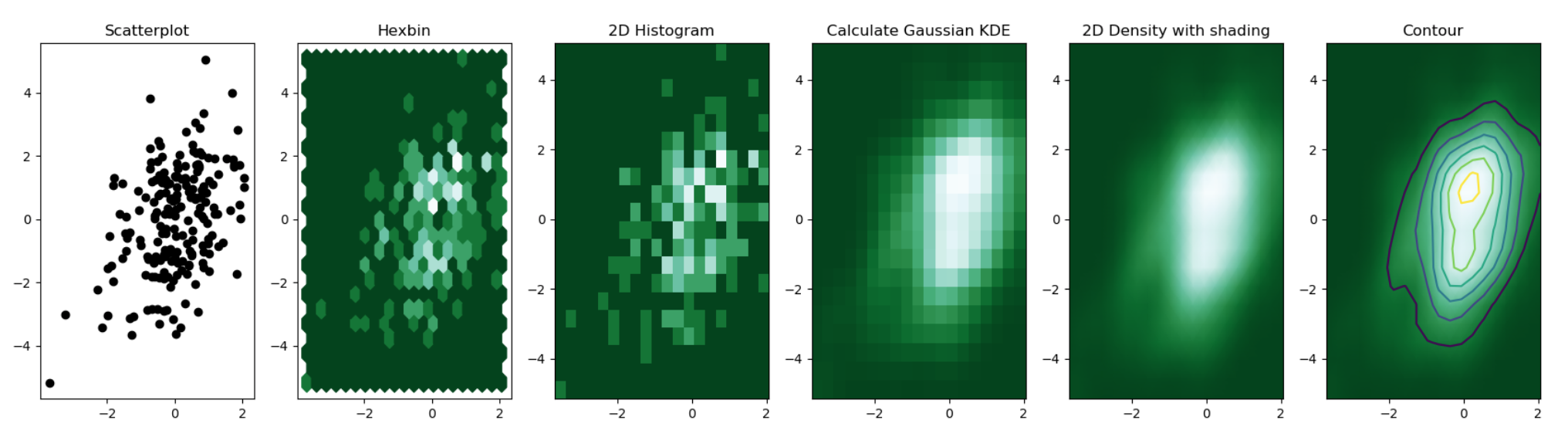# Density 2d

A 2d density chart displays the relationship between 2 numeric variables. One is represented on the X axis, the other on the Y axis, like for a scatterplot. Then, the number of observations within a particular area of the 2D space is counted and represented by a color gradient. Several types of 2d density chart exist:Different types of 2d density chart. Source

2d histogram with `geom_bin2d()`

This is the two dimension version of the classic histogram. The plot area is split in a multitude of small squares, the number of points in each square is represented by its color.

Hexbin chart with `geom_hex()`

Very similar to the 2d histogram above, but the plot area is split in a multitude of hexagons instead of squares.

2d distribution with `stat_density_2d`

Like it is possible to plot a density chart instead of a histogram to represent a distribution, it is possible to make a 2d density plot. Several variations are available using `ggplot2`:

Other examples

Related chart types